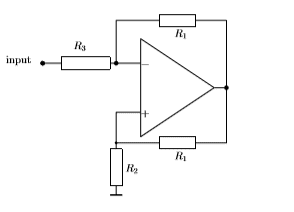# Circuit input impedance with ground in an OP Amp circuit

• Engineering
bobg123
Homework Statement:
Find input impedance of the circuit shown. Calculate impedance between the input terminal and the ”ground”. Two resistors R1 have equal resistance.
Relevant Equations:
V-=V+
V=IR
V_out=-R1/R3 Vin
V_out=R1/R2+1 Vin
I've been given the following circuit and have been asked to find the input impedance and the impedance between the input terminal and ground. I've never encountered an operational amplifier configured like this.I know that the voltages at the - and + terminals of the op amp are ideally equal. In a normal inverting/non inverting op amp, I've been told the voltages at those points are zero. Is this still the case? If so, what is the output voltage? I'm having trouble conceptually understanding what this particular circuit does.

I'm not sure how to approach this problem, but I've tried separating the circuit into an inverting and non-inverting circuit. For the inverting circuit, I know V_out=-V_in*R1/R3. This gives me V(-)=V_in+V_in/R_3. For the noninverting, I know V_out=(R1/R2+1)V_in. The voltage at V(+) should be equal to V(-), giving V_in+V_in/R_3=V(R1)-V(R2)=R1/R2V_in+V_in-V(R2). Some rearrangement gives me V_in(1/R3-R1/R2)=-V(R2). Is this the right way of approaching the problem? Where do I go from here to calculate the impedance?

Staff Emeritus
I know that the voltages at the - and + terminals of the op amp are ideally equal.
That's one assumption you need to make, the other is that negligible current is drawn into those op-amp inputs. It would be wrong to think there are any virtual grounds here.

As always, input impedance = input voltage ÷ input current
and for this circuit, input current = the current in R3.

LvW
Calculate ther gain of the circuit.
Then, it will not be a problem to find the current through the chain R3..R1.

Gold Member
I've been given the following circuit and have been asked to find the input impedance and the impedance between the input terminal and ground. I've never encountered an operational amplifier configured like this.
View attachment 241071
I know that the voltages at the - and + terminals of the op amp are ideally equal. In a normal inverting/non inverting op amp, I've been told the voltages at those points are zero. Is this still the case? If so, what is the output voltage? I'm having trouble conceptually understanding what this particular circuit does.

I'm not sure how to approach this problem, but I've tried separating the circuit into an inverting and non-inverting circuit. For the inverting circuit, I know V_out=-V_in*R1/R3. This gives me V(-)=V_in+V_in/R_3. For the noninverting, I know V_out=(R1/R2+1)V_in. The voltage at V(+) should be equal to V(-), giving V_in+V_in/R_3=V(R1)-V(R2)=R1/R2V_in+V_in-V(R2). Some rearrangement gives me V_in(1/R3-R1/R2)=-V(R2). Is this the right way of approaching the problem? Where do I go from here to calculate the impedance?

Are you still working on this problem?

One problem I see is that in this:

V_in+V_in/R_3=V(R1)-V(R2)=R1/R2V_in+V_in-V(R2)

You are equating a sum of a voltage (red) and a current (blue) to a voltage (green). That's not permissible.

LvW
Quote: I know V_out=-V_in*R1/R3

No - that is not correct.
This simple expression is valid in case the feedback path contains these two resistors only (negative feedback).
However - in addition, we have positive feedback via R1 and R2.
Note, that the above simple gain expression results from a general formula for feedback circuits (and idealized open-loop gain): A=-Hf/Hr.
Both expressions Hf and Hr consist of simple voltage dividers and are defined as follows
(Vd=Diff. voltage at the opamps input):
* Forward damping: Hf=Vd/Vin for Vout=0
* Return damping (feedback factor): Hr=Vd/Vout for Vin=0.

This general formula is to be used also for the given circuit.